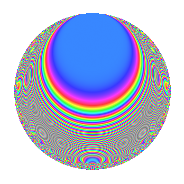# Properties

 Label 252.2.beLevel 252 Weight 2 Character orbit be Rep. character $$\chi_{252}(107,\cdot)$$ Character field $$\Q(\zeta_{6})$$ Dimension 32 Newform subspaces 1 Sturm bound 96 Trace bound 0

# Related objects

## Defining parameters

 Level: $$N$$ = $$252 = 2^{2} \cdot 3^{2} \cdot 7$$ Weight: $$k$$ = $$2$$ Character orbit: $$[\chi]$$ = 252.be (of order $$6$$ and degree $$2$$) Character conductor: $$\operatorname{cond}(\chi)$$ = $$84$$ Character field: $$\Q(\zeta_{6})$$ Newform subspaces: $$1$$ Sturm bound: $$96$$ Trace bound: $$0$$

## Dimensions

The following table gives the dimensions of various subspaces of $$M_{2}(252, [\chi])$$.

Total New Old
Modular forms 112 32 80
Cusp forms 80 32 48
Eisenstein series 32 0 32

## Trace form

 $$32q + O(q^{10})$$ $$32q + 16q^{13} + 4q^{16} - 32q^{22} + 24q^{25} - 44q^{28} - 16q^{34} + 8q^{37} - 52q^{40} - 24q^{46} - 16q^{49} - 52q^{52} - 12q^{58} - 16q^{61} + 120q^{64} + 60q^{70} - 8q^{73} + 72q^{76} + 68q^{82} - 32q^{85} + 44q^{88} + 60q^{94} - 176q^{97} + O(q^{100})$$

## Decomposition of $$S_{2}^{\mathrm{new}}(252, [\chi])$$ into newform subspaces

Label Dim. $$A$$ Field CM Traces $q$-expansion
$$a_2$$ $$a_3$$ $$a_5$$ $$a_7$$
252.2.be.a $$32$$ $$2.012$$ None $$0$$ $$0$$ $$0$$ $$0$$

## Decomposition of $$S_{2}^{\mathrm{old}}(252, [\chi])$$ into lower level spaces

$$S_{2}^{\mathrm{old}}(252, [\chi]) \cong$$ $$S_{2}^{\mathrm{new}}(84, [\chi])$$$$^{\oplus 2}$$

## Hecke Characteristic Polynomials

There are no characteristic polynomials of Hecke operators in the database